• +91 9971497814
• info@interviewmaterial.com

# Arithmetic Progressions Ex-5.2 Interview Questions Answers

### Related Subjects

Question 1 :

Fill in the blanksin the following table, given that a is the first term, d thecommon difference and an the nth termof the A.P.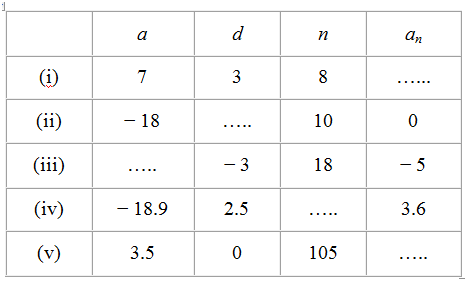Solution

(i) Given,

First term, a = 7

Common difference, d = 3

Number of terms, n = 8,

We have to find the nth term, an =?

As we know, for an A.P.,

an = a+(n−1)d

Putting the values,

=> 7+(8 −1) 3

=> 7+(7) 3

=> 7+21 = 28

Hence, an = 28

Solution

(ii) Given,

First term, a = -18

Common difference, d = ?

Number of terms, n = 10

Nth term, an = 0

As we know, for an A.P.,

an = a+(n−1)d

Putting the values,

0 = − 18 +(10−1)d

18 = 9d

d = 18/9 = 2

Hence, common difference, =2

Solution

(iii) Given,

First term, a = ?

Common difference, d = -3

Number of terms, n = 18

Nth term, an = -5

As we know, for an A.P.,

an = a+(n−1)d

Putting the values,

−5 = a+(18−1) (−3)

−5 = a+(17) (−3)

−5 = a−51

a = 51−5 = 46

Hence, a = 46

Solution

(iv) Given,

First term, a = -18.9

Common difference, d = 2.5

Number of terms, n = ?

Nth term, an = 3.6

As we know, for an A.P.,

an = a +(n −1)d

Putting the values,

3.6 = − 18.9+(n −1)2.5

3.6 + 18.9 = (n−1)2.5

22.5 = (n−1)2.5

(n – 1) = 22.5/2.5

n – 1 = 9

n = 10

Hence, n = 10

Solution

(v) Given,

First term, a = 3.5

Common difference, d = 0

Number of terms, n = 105

Nth term, an = ?

As we know, for an A.P.,

an = a+(n −1)d

Putting the values,

an = 3.5+(105−1) 0

an = 3.5+104×0

an = 3.5

Hence, an = 3.5

Question 2 : Choose the correct choice in the following and justify:

(i) 30th term of the AP: 10, 7, 4, …, is
(a) 97              (b) 77              (c) -77               (d) -87

(ii) 11th term of the AP: -3, −12 , 2, …, is
(a) 28              (b) 22               (c) -38              (d) -48
Solution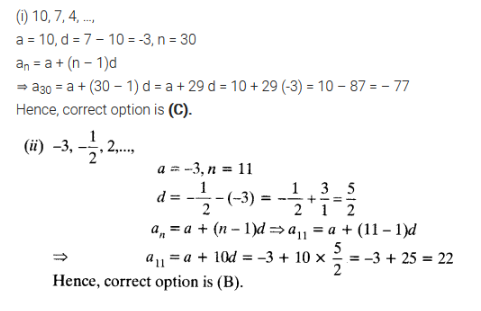Question 3 : In the following APs, find the missing terms in the boxes

Answer 3 :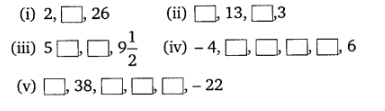Solution: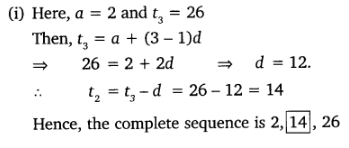Solution: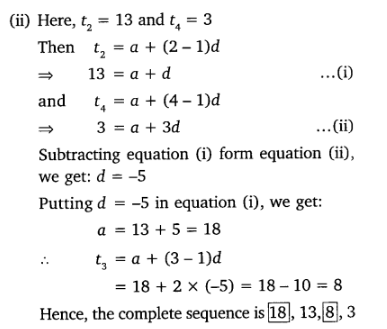Solution: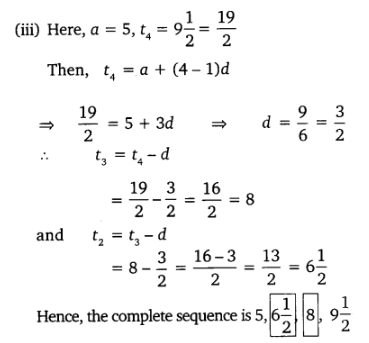Solution: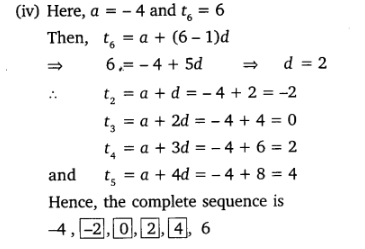Solution: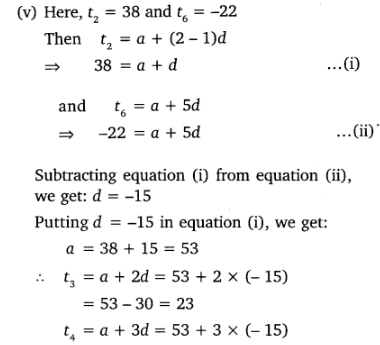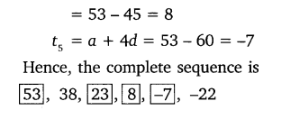Question 4 : Which term of the A.P. 3, 8, 13, 18, … is 78?

Given the A.P. series as3, 8, 13, 18, …

First term, a = 3

Common difference, d = a2 − a1 =8 − 3 = 5

Let the nth termof given A.P. be 78. Now as we know,

an = a+(n−1)d

Therefore,

78 = 3+(n −1)5

75 = (n−1)5

(n−1) = 15

n = 16

Hence, 16th term of this A.P.is 78.

Question 5 : Find the number of terms in each of the following A.P.

(i) 7, 13, 19, …,205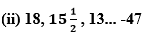Solutions:

(i) Given, 7, 13,19, …, 205 is the A.P

Therefore

First term, a = 7

Common difference, d = a2 − a1 =13 − 7 = 6

Let there are n terms in thisA.P.

an = 205

As we know, for an A.P.,

an = a + (n − 1) d

Therefore, 205 = 7 + (− 1) 6

198 = (n − 1) 6

33 = (n − 1)

n = 34

Therefore, this given series has 34 terms init.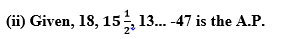First term, a = 18

Common difference, d = a2-a=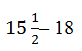d = (31-36)/2 = -5/2

Let there are n terms in this A.P.

an = 205

As we know, for an A.P.,

an = a+(n−1)d

-47 = 18+(n-1)(-5/2)

-47-18 = (n-1)(-5/2)

-65 = (n-1)(-5/2)

(n-1) = -130/-5

(n-1) = 26

n = 27

Therefore, this given A.P. has 27 terms in it.

Question 6 :

Check whether -150 is a term of the A.P. 11, 8, 5, 2, …

For the given series, A.P. 11, 8, 5, 2..

First term, a = 11

Common difference, d = a2a1 =8−11 = −3

Let −150 be the nth termof this A.P.

As we know, for an A.P.,

an = a+(n−1)d

-150 = 11+(n -1)(-3)

-150 = 11-3n +3

-164 = -3n

n = 164/3

Clearly, n is not an integerbut a fraction.

Therefore, – 150 is not a term of this A.P.

Question 7 :

Find the 31_st term of an A.P. whose 11_th term is 38 and the 16_th term is 73.

Given that,

11th term, a11 =38

and 16th term, a16 =73

We know that,

an = a+(n−1)d

a11 = a+(11−1)d

38 = a+10d ………………………………. (i)

In the same way,

a16 = a +(16−1)d

73 = a+15d ………………………………………… (ii)

On subtracting equation (i) from (ii),we get

35 = 5d

d = 7

From equation (i), we can write,

38 = a+10×(7)

38 − 70 = a

a = −32

a31 = a +(31−1) d

= − 32 + 30 (7)

= − 32 + 210

= 178

Hence, 31st term is 178.

Question 8 :

An A.P. consists of 50 terms of which 3_rd term is 12 and the last term is 106. Find the 29_th term.

Given that,

3rd term, a3 =12

50th term, a50 =106

We know that,

an = a+(n−1)d

a3 = a+(3−1)d

12 = a+2d ……………………………. (i)

In the same way,

a50 a+(50−1)d

106 = a+49d …………………………. (ii)

On subtracting equation (i) from (ii), we get

94 = 47d

d = 2 = commondifference

From equation (i), we can write now,

12 = a+2(2)

a = 12−4 = 8

a29 = a+(29−1) d

a29 = 8+(28)2

a29 = 8+56 = 64

Therefore, 29th term is 64.

Question 9 :  If the 3_rd and the 9_th terms of an A.P. are 4 and − 8 respectively. Which term of this A.P. is zero.

Given that,

3rd term, a3 = 4

and 9th term, a9 = −8

We know that,

an = a+(n−1)d

Therefore,

a3 = a+(3−1)d

4 = a+2d ……………………………………… (i)

a9 = a+(9−1)d

−8 = a+8d ………………………………………………… (ii)

On subtracting equation (i) from (ii), we will get here,

−12 = 6d

d = −2

From equation (i), we can write,

4 = a+2(−2)

4 = a−4

a = 8

Let nth term ofthis A.P. be zero.

aa+(n−1)d

0 = 8+(n−1)(−2)

0 = 8−2n+2

2= 10

n = 5

Hence, 5th termof this A.P. is 0.

Question 10 : The 17th term of an AP exceeds its 10th term by 7. Find the common difference.

Answer 10 :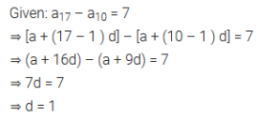Todays Deals### Arithmetic Progressions Ex-5.2 Contributorskrishan

Name:
Email:

# Latest News# 9000 interview questions in different categories